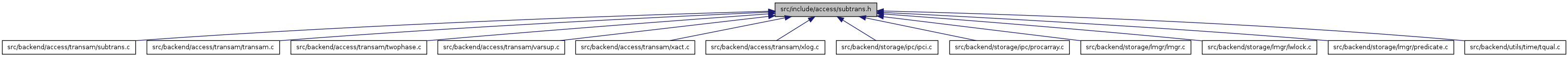# subtrans.h File Reference

This graph shows which files directly or indirectly include this file:Go to the source code of this file.

## Defines

#define NUM_SUBTRANS_BUFFERS   32

## Functions

void SubTransSetParent (TransactionId xid, TransactionId parent, bool overwriteOK)
TransactionId SubTransGetParent (TransactionId xid)
TransactionId SubTransGetTopmostTransaction (TransactionId xid)
Size SUBTRANSShmemSize (void)
void SUBTRANSShmemInit (void)
void BootStrapSUBTRANS (void)
void StartupSUBTRANS (TransactionId oldestActiveXID)
void ShutdownSUBTRANS (void)
void CheckPointSUBTRANS (void)
void TruncateSUBTRANS (TransactionId oldestXact)

## Define Documentation

 #define NUM_SUBTRANS_BUFFERS   32

Definition at line 15 of file subtrans.h.

Referenced by SUBTRANSShmemInit(), and SUBTRANSShmemSize().

## Function Documentation

 void BootStrapSUBTRANS ( void )

Definition at line 198 of file subtrans.c.

Referenced by BootStrapXLOG().

```{
int         slotno;

LWLockAcquire(SubtransControlLock, LW_EXCLUSIVE);

/* Create and zero the first page of the subtrans log */
slotno = ZeroSUBTRANSPage(0);

/* Make sure it's written out */
SimpleLruWritePage(SubTransCtl, slotno);
Assert(!SubTransCtl->shared->page_dirty[slotno]);

LWLockRelease(SubtransControlLock);
}
```

 void CheckPointSUBTRANS ( void )

Definition at line 283 of file subtrans.c.

References SimpleLruFlush(), and SubTransCtl.

Referenced by CheckPointGuts().

```{
/*
* Flush dirty SUBTRANS pages to disk
*
* This is not actually necessary from a correctness point of view. We do
* it merely to improve the odds that writing of dirty pages is done by
* the checkpoint process and not by backends.
*/
TRACE_POSTGRESQL_SUBTRANS_CHECKPOINT_START(true);
SimpleLruFlush(SubTransCtl, true);
TRACE_POSTGRESQL_SUBTRANS_CHECKPOINT_DONE(true);
}
```

 void ExtendSUBTRANS ( TransactionId newestXact )

Definition at line 307 of file subtrans.c.

Referenced by GetNewTransactionId(), and RecordKnownAssignedTransactionIds().

```{
int         pageno;

/*
* No work except at first XID of a page.  But beware: just after
* wraparound, the first XID of page zero is FirstNormalTransactionId.
*/
return;

LWLockAcquire(SubtransControlLock, LW_EXCLUSIVE);

/* Zero the page */
ZeroSUBTRANSPage(pageno);

LWLockRelease(SubtransControlLock);
}
```

 void ShutdownSUBTRANS ( void )

Definition at line 266 of file subtrans.c.

References SimpleLruFlush(), and SubTransCtl.

Referenced by ShutdownXLOG().

```{
/*
* Flush dirty SUBTRANS pages to disk
*
* This is not actually necessary from a correctness point of view. We do
* it merely as a debugging aid.
*/
TRACE_POSTGRESQL_SUBTRANS_CHECKPOINT_START(false);
SimpleLruFlush(SubTransCtl, false);
TRACE_POSTGRESQL_SUBTRANS_CHECKPOINT_DONE(false);
}
```

 void StartupSUBTRANS ( TransactionId oldestActiveXID )

Definition at line 236 of file subtrans.c.

Referenced by StartupXLOG().

```{
int         startPage;
int         endPage;

/*
* Since we don't expect pg_subtrans to be valid across crashes, we
* initialize the currently-active page(s) to zeroes during startup.
* Whenever we advance into a new page, ExtendSUBTRANS will likewise zero
* the new page without regard to whatever was previously on disk.
*/
LWLockAcquire(SubtransControlLock, LW_EXCLUSIVE);

startPage = TransactionIdToPage(oldestActiveXID);
endPage = TransactionIdToPage(ShmemVariableCache->nextXid);

while (startPage != endPage)
{
(void) ZeroSUBTRANSPage(startPage);
startPage++;
}
(void) ZeroSUBTRANSPage(startPage);

LWLockRelease(SubtransControlLock);
}
```

 TransactionId SubTransGetParent ( TransactionId xid )

Definition at line 105 of file subtrans.c.

```{
int         pageno = TransactionIdToPage(xid);
int         entryno = TransactionIdToEntry(xid);
int         slotno;
TransactionId *ptr;
TransactionId parent;

/* Can't ask about stuff that might not be around anymore */
Assert(TransactionIdFollowsOrEquals(xid, TransactionXmin));

/* Bootstrap and frozen XIDs have no parent */
if (!TransactionIdIsNormal(xid))
return InvalidTransactionId;

ptr = (TransactionId *) SubTransCtl->shared->page_buffer[slotno];
ptr += entryno;

parent = *ptr;

LWLockRelease(SubtransControlLock);

return parent;
}
```

 TransactionId SubTransGetTopmostTransaction ( TransactionId xid )

Definition at line 146 of file subtrans.c.

```{
TransactionId parentXid = xid,
previousXid = xid;

/* Can't ask about stuff that might not be around anymore */
Assert(TransactionIdFollowsOrEquals(xid, TransactionXmin));

while (TransactionIdIsValid(parentXid))
{
previousXid = parentXid;
if (TransactionIdPrecedes(parentXid, TransactionXmin))
break;
parentXid = SubTransGetParent(parentXid);
}

Assert(TransactionIdIsValid(previousXid));

return previousXid;
}
```

 void SubTransSetParent ( TransactionId xid, TransactionId parent, bool overwriteOK )

Definition at line 75 of file subtrans.c.

```{
int         pageno = TransactionIdToPage(xid);
int         entryno = TransactionIdToEntry(xid);
int         slotno;
TransactionId *ptr;

Assert(TransactionIdIsValid(parent));

LWLockAcquire(SubtransControlLock, LW_EXCLUSIVE);

slotno = SimpleLruReadPage(SubTransCtl, pageno, true, xid);
ptr = (TransactionId *) SubTransCtl->shared->page_buffer[slotno];
ptr += entryno;

/* Current state should be 0 */
Assert(*ptr == InvalidTransactionId ||
(*ptr == parent && overwriteOK));

*ptr = parent;

SubTransCtl->shared->page_dirty[slotno] = true;

LWLockRelease(SubtransControlLock);
}
```

 void SUBTRANSShmemInit ( void )

Definition at line 178 of file subtrans.c.

References NUM_SUBTRANS_BUFFERS, SimpleLruInit(), SubtransControlLock, and SubTransCtl.

Referenced by CreateSharedMemoryAndSemaphores().

```{
SubTransCtl->PagePrecedes = SubTransPagePrecedes;
SimpleLruInit(SubTransCtl, "SUBTRANS Ctl", NUM_SUBTRANS_BUFFERS, 0,
SubtransControlLock, "pg_subtrans");
/* Override default assumption that writes should be fsync'd */
SubTransCtl->do_fsync = false;
}
```

 Size SUBTRANSShmemSize ( void )

Definition at line 172 of file subtrans.c.

References NUM_SUBTRANS_BUFFERS, and SimpleLruShmemSize().

Referenced by CreateSharedMemoryAndSemaphores().

```{
return SimpleLruShmemSize(NUM_SUBTRANS_BUFFERS, 0);
}
```

 void TruncateSUBTRANS ( TransactionId oldestXact )

Definition at line 337 of file subtrans.c.

References SimpleLruTruncate(), SubTransCtl, and TransactionIdToPage.

Referenced by CreateCheckPoint(), and CreateRestartPoint().

```{
int         cutoffPage;

/*
* The cutoff point is the start of the segment containing oldestXact. We
* pass the *page* containing oldestXact to SimpleLruTruncate.
*/
cutoffPage = TransactionIdToPage(oldestXact);

SimpleLruTruncate(SubTransCtl, cutoffPage);
}
```